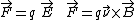Aharonov-Bohm effect

Also found in: Wikipedia.

Aharonov-Bohm effect

The predicted effect of an electromagnetic vector or scalar potential in electronic interference phenomena, in the absence of electric or magnetic fields on the electrons.

The fundamental equations of motion for a charged object are usually expressed in terms of the magnetic field and the electric field. The force on a charged particle can be conveniently written as in the equations below, where q is theparticle's charge, is its velocity, and the symbol × represents the vector product. Associated with is a scalar potential V defined at any point as the work W necessary to move a charge from minus infinity to that point, V = W/q. Generally, only the difference in potentials between two points matters in classical physics, and this potential difference can be used in computing the electric field. Similarly, associated with is a vector potential, a convenient mathematical aid for calculating the magnetic field. See Electric field, Potentials

In quantum mechanics, however, the basic equations that describe the motion of all objects contain and V directly, and they cannot be simply eliminated. Nonetheless, it was initially believed that these potentials had no independent significance. In 1959, Y. Aharonov and D. Bohm discovered that both the scalar and vector potentials should play a major role in quantum mechanics. They proposed two electron interference experiments in which some of the electron properties would be sensitive to changes of or V, even when there were no electric or magnetic fields present on the charged particles. The absence of and means that classically there are no forces acting on the particles, but quantum-mechanically it is still possible to change the properties of the electron. These counterintuitive predictions are known as the Aharonov-Bohm effect.

Surprisingly, the Aharonov-Bohm effect plays an important role in understanding the properties of electrical circuits whose wires or transistors are smaller than a few micrometers. The electrical resistance in a wire loop oscillates periodically as the magnetic flux threading the loop is increased, with a period of h/e (where h is Planck's constant and e is the charge of the electron), the normal-metal flux quantum. In single wires, the electrical resistance fluctuates randomly as a function of magnetic flux. Both these observations, which were made possible by advances in the technology for fabricating small samples, reflect an Aharonov-Bohm effect. They have opened up a new field of condensed-matter physics because they are a signature that the electrical properties are dominated by quantum-mechanical behavior of the electrons, and that the rules of the classical physics are no longer operative. See Quantum mechanics

Aharonov-Bohm effect

[‚ä·hä′rō‚nȯf ′bām i‚fekt]
(quantum mechanics)
An effect manifested when a beam of electrons is split into two beams that travel in opposite directions around a region containing magnetic flux and are then recombined, whereby the intensity of the resulting beam oscillates periodically as the enclosed magnetic field is changed.
References in periodicals archive ?
The Aharonov-Bohm effect (1959) may even show a relation to the theory of everything (TOE), where potentials are extended to "gauge fields" and regarded as a fundamental physical quantity.
Among his topics are re-inflating the conception of scientific representation, gauge gravity and the unification of natural forces, spontaneous symmetry breaking and chance in a classical world, a possible unified understanding of probabilistic objects, and the Aharonov-Bohm effect and the reality of wave packets.
This quantum effect is known as the Aharonov-Bohm effect (AB effect) ().
A toroidal magnetic field has been used in an experimental verification of the Aharonov-Bohm effect [1,2].
Notably, the Aharonov-Bohm effect was demonstrated using electron beam holography generated by a Hitachi FE-TEM (Field Emission-Transmission Electron Microscope) fitted with an FE electron beam source.
g] and stabilization of the modulus when a magnetic field is applied, attributes to a unique characteristic of MWCNTs known as the Aharonov-Bohm effect .
The Aharonov-Bohm effect, and related non-local topological effects involving the Berry Phase, can be derived directly from Cauchy's Residue Theorem applied to a gauge-potential (eg.
He begins with manifolds, tensors and exterior forms and progresses to such topics as the integration of differential forms and the Lie derivative, the Poincare Lemma and potentials, Monkowski space, covariant differentiation and curvature, relativity, Betti numbers and De Rham's theorem, harmonic forms, the Aharonov-Bohm effect, and Yang- Mills fields.
It is widely agreed that the subsequent experimental detection of the Aharonov-Bohm effect discredited the familiar way of understanding electromagnetism.
With a wealth of examples ranging from tooth fairies to dinosaur fights, from the Aharonov-Bohm effect to Schrodinger's equation, he mounts a literate argument for realism.
As in the case of the Aharonov-Bohm effect, an interference experiment is a good place to look for evidence, this time with neutrons substituted for electrons and a charged electrode for the solenoid.
Site: Follow: Share:
Open / Close